# Quiz 36: Extending the Analysis of Aggregate Supply

Business

Remember that the basic equation of monetarism is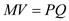Where " M " refers to the supply of money, " V " refers to the velocity of money (the number of times a year on average a dollar is circulated in the economy), " P " is the price level, and " Q " is the physical volume of all goods and services produced. Note that PQ is the same as the GDP of the economy. We are given that the nominal GDP of the economy is \$336 billion, which means that PQ is \$96 billion. Further, we are given that the money supply of the economy is \$96 billion. Substituting these numbers into the equation of monetarism, we have: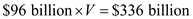If we divided \$96 billion to both sides, we get:Hence, the velocity of money in the economy iS3.5 If money supply falls by \$20 billion, we see that the total spending of the economy will reduce by \$70 billion. This is because a \$20 billion decrease in money supply will be multiplied by the velocity of 3.5 to get the total decrease of spending that resulted from the \$20 billion, as each dollar on average is circulateD3.5 times a year in the economy, so decreasing the money supply by \$20 billion will cause a decrease of \$70 billion of spending that year. Since spending decreased by \$70 billion (since the monetarist perspective is that the velocity of money is stable), this must mean that the right hand side of the equation, PQ , must also decrease by \$70 billion in order for equilibrium to be achieved. Therefore, the nominal GDP must fall by \$70 billion for the economy to be back in equilibrium.

Since both input and output prices are fixed in the economy, this must mean that we are looking at the immediate-short-run of the economy (where prices are not flexible and are constant). Thus, the aggregate supply curve will be perfectly horizontal, which shows that prices do not change. If the aggregate demand decreases in this situation, we see that price will remain unchanged, but the real output of the economy will fall. This is simply due to lower demand in the economy, which will shift aggregate demand to the left. The intersection point between the aggregate supply and the new aggregate demand will be at a lower real output level. If input prices are fixed but output prices are not, we are now looking at the economy in the short run. The aggregate supply is upward sloping, signifying that higher output prices will mean suppliers would want to supply more, thus increasing aggregate supply and real output. If aggregate demand decreases, we see that both the price level and real output will fall. The new intersection between the aggregate supply and aggregate demand curve will occur at a point such that the price level and real output will be lower than it was before. If both input and output prices are flexible, then we are looking at the long run of the economy. In the long run, the aggregate supply curve is perfectly vertical, meaning that prices are completely flexible. If the aggregate demand decreases, real output will not change at all but the price level will decrease in the economy. Notice that with a perfectly vertical aggregate supply curve, the intersection between the aggregate demand and aggregate supply will always happen at the same level of real output. Shifts in the aggregate demand curve will, however, change the price level.

(a) We are given the following information in year 1 of an economy: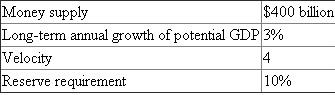To find nominal GDP, we can use the basic equation of monetarism (or the equation of exchange):Here, " M " refers to the supply of money, " V " refers to the velocity of money (the number of times a year on average a dollar is circulated in the economy), " P " is the price level, and " I " is the physical volume of all goods and services produced. Note that PQ is the same as the GDP of the economy. Since we are not given P or Q , we must use MV to find what PQ , or nominal GDP, will be. We are given that M , or the money supply, is \$400 billion, and that V , the velocity, is 4. Therefore,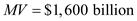. Note that in equilibrium,, so it must also be that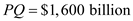. In other words, nominal GDP in year 1 is \$1,600 billion. (b) Since the economy is growing at 3% per year, it must mean that the money supply must also grow at 3% per year (since velocity is assumed to be constant). We are given that in year 1, money supply is \$400 billion. Therefore, to grow at 3%, this must mean money supply must increase byTo increase money supply by \$12 billion, the Federal Reserve must buy securities from banks or the public between yearS1 anD2. In buying securities, the Federal Reserve will inject cash into the economy and own securities. The increase in cash will increase the money supply, thus satisfying the monetary rule.